Скачать презентацию 1 Choosing independent variables • The main

l2_choosing_iv_3_methods.ppt

• Размер: 725.5 Кб
• Количество слайдов: 38

## Описание презентации 1 Choosing independent variables • The main по слайдам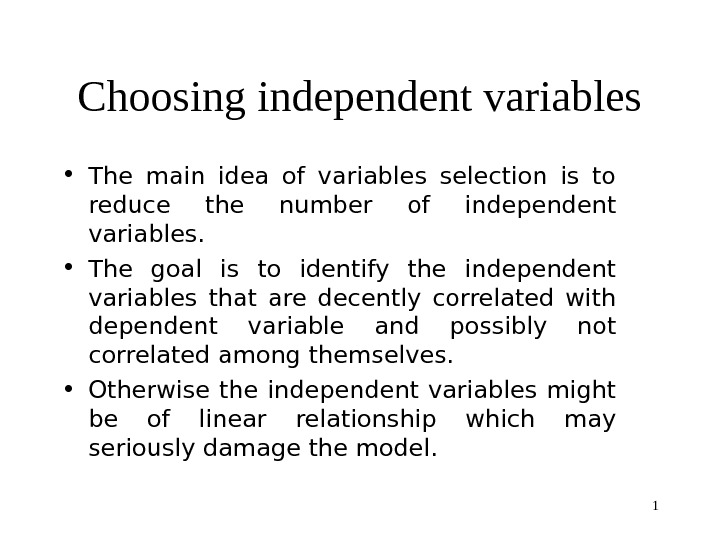1 Choosing independent variables • The main idea of variables selection is to reduce the number of independent variables. • The goal is to identify the independent variables that are decently correlated with dependent variable and possibly not correlated among themselves. • Otherwise the independent variables might be of linear relationship which may seriously damage the model.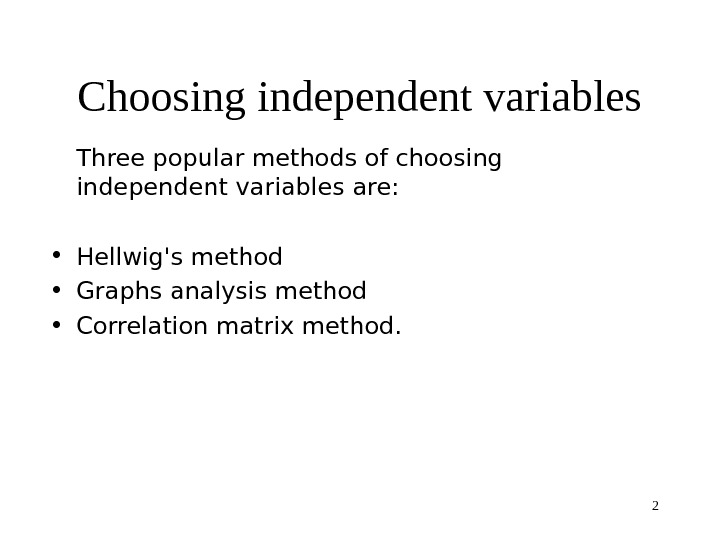2 Choosing independent variables T hree popular methods of choosing independent variables are: • Hellwig’s method • Graphs analysis method • Correlation matrix method.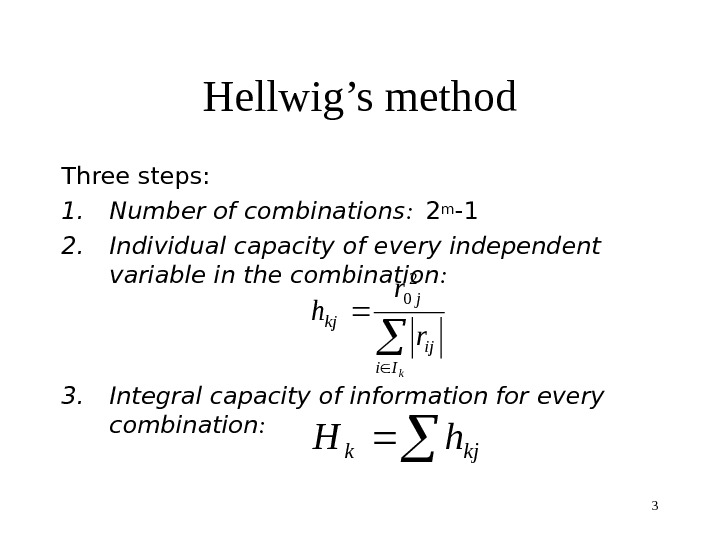3 Hellwig’s method Three steps: 1. Number of combinations : 2 m -1 2. Individual capacity of every independent variable in the combination : 3. Integral capacity of information for every combination : k. Ii ij j kj r r h 2 0 kjkh. H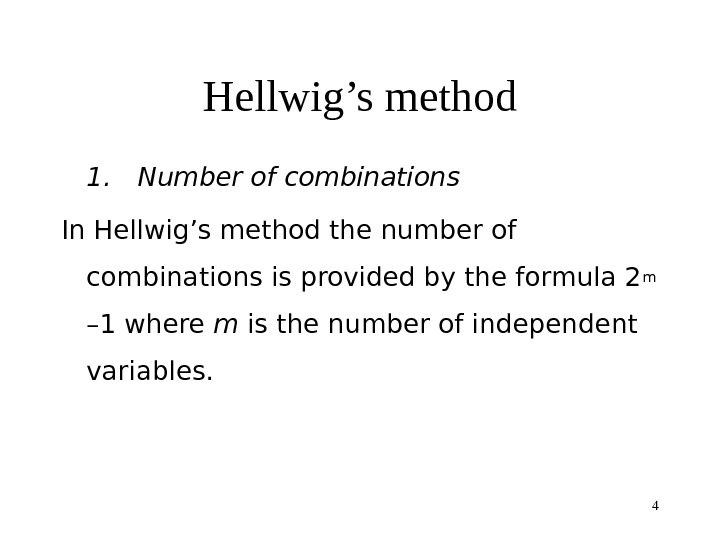4 Hellwig’s method 1. Number of combinations In Hellwig’s method the number of combinations is provided by the formula 2 m – 1 where m is the number of independent variables.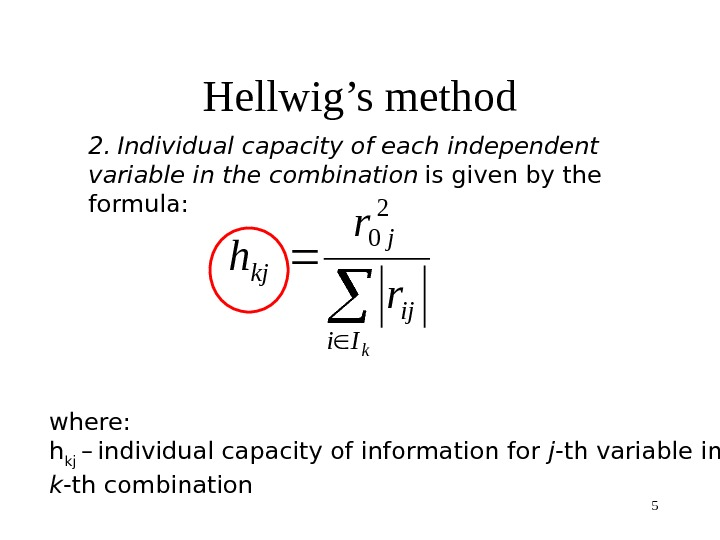5 Hellwig’s method 2. Individual capacity of each independent variable in the combination is given by the formula: k. Ii ij j kj r r h 2 0 where: h kj – individual capacity of information for j -th variable in k -th combination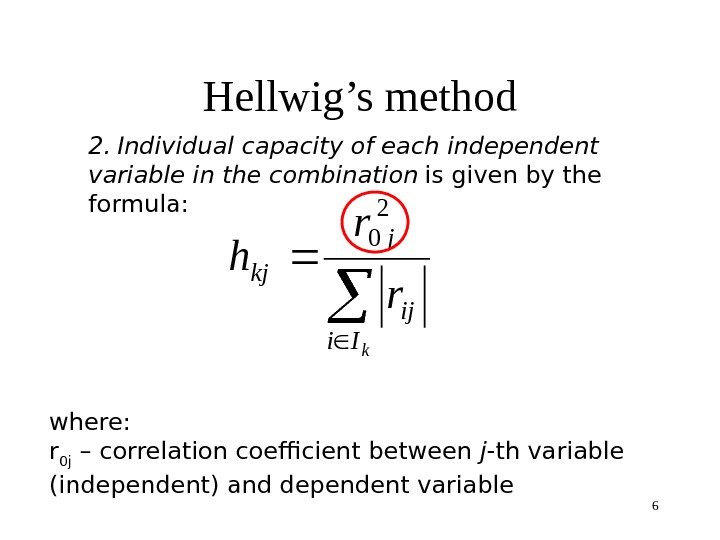6 Hellwig’s method 2. Individual capacity of each independent variable in the combination is given by the formula: k. Ii ij j kj r r h 2 0 where: r 0 j – correlation coefficient between j -th variable (independent) and dependent variable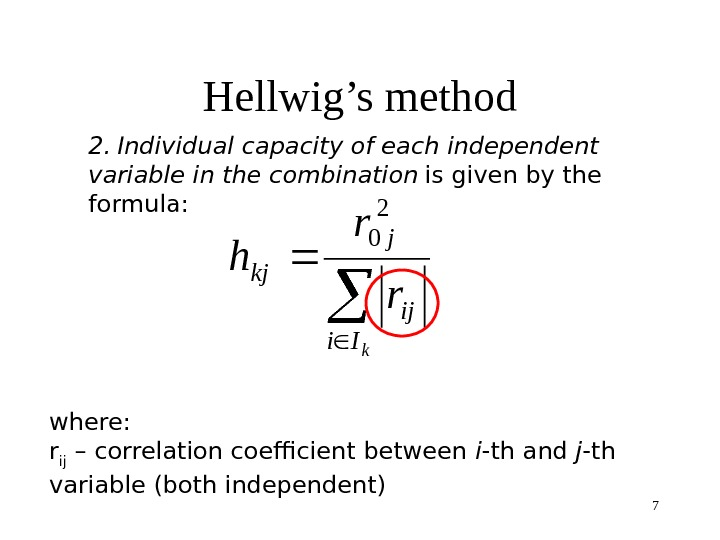7 Hellwig’s method 2. Individual capacity of each independent variable in the combination is given by the formula: k. Ii ij j kj r r h 2 0 where: r ij – correlation coefficient between i -th and j -th variable (both independent)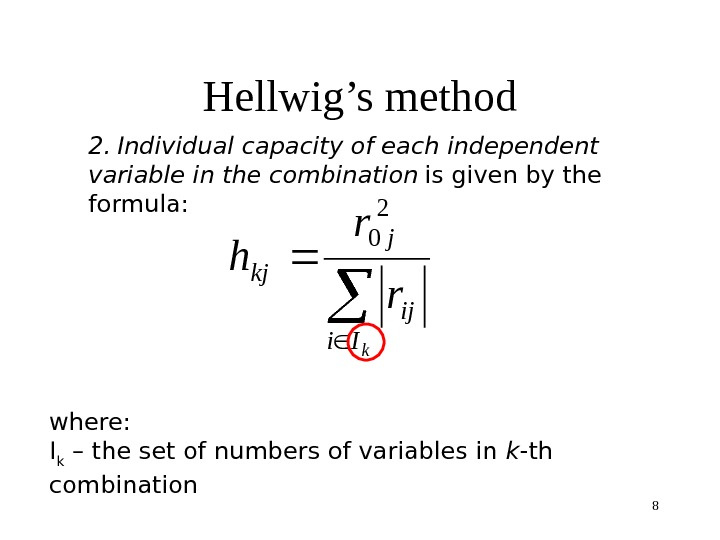8 Hellwig’s method 2. Individual capacity of each independent variable in the combination is given by the formula: k. Ii ij j kj r r h 2 0 where: I k – the set of numbers of variables in k -th combination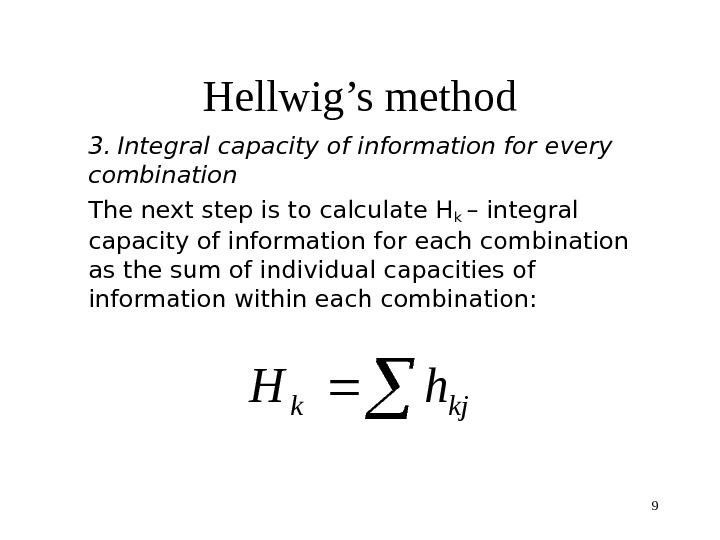9 Hellwig’s method 3. Integral capacity of information for every combination The next step is to calculate Hk – integral capacity of information for each combination as the sum of individual capacities of information within each combination: kjk h. H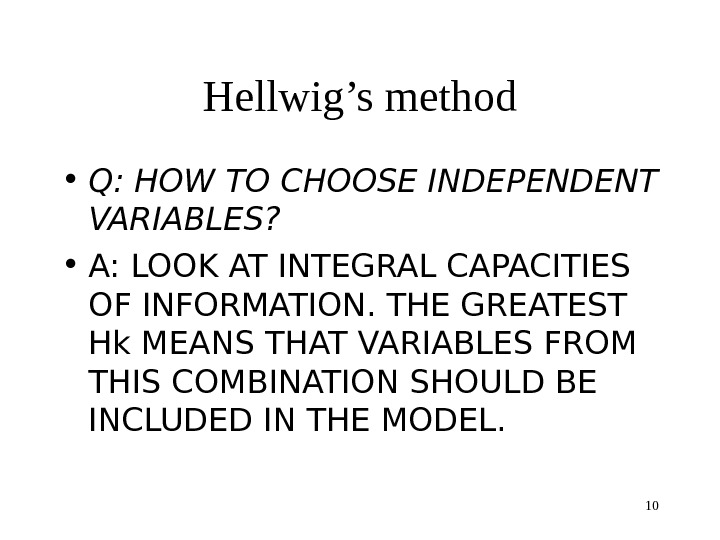10 Hellwig’s method • Q: HOW TO CHOOSE INDEPENDENT VARIABLES? • A: LOOK AT INTEGRAL CAPACITIES OF INFORMATION. THE GREATEST Hk MEANS THAT VARIABLES FROM THIS COMBINATION SHOULD BE INCLUDED IN THE MODEL.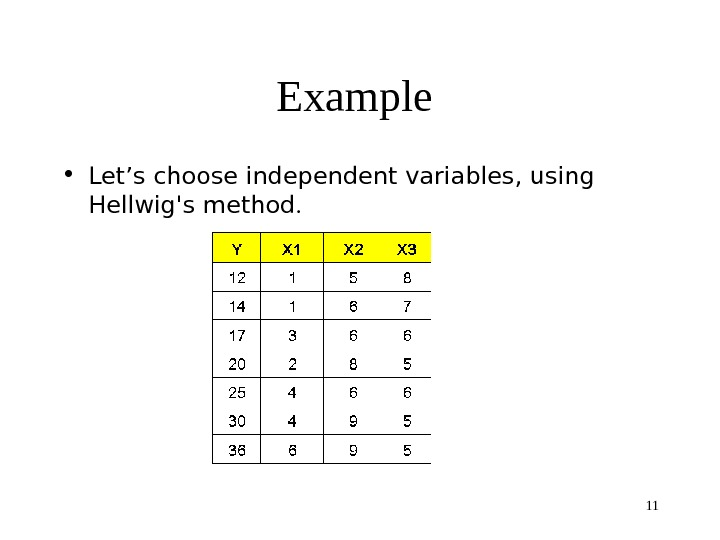11 Example • Let’s choose independent variables, using Hellwig’s method.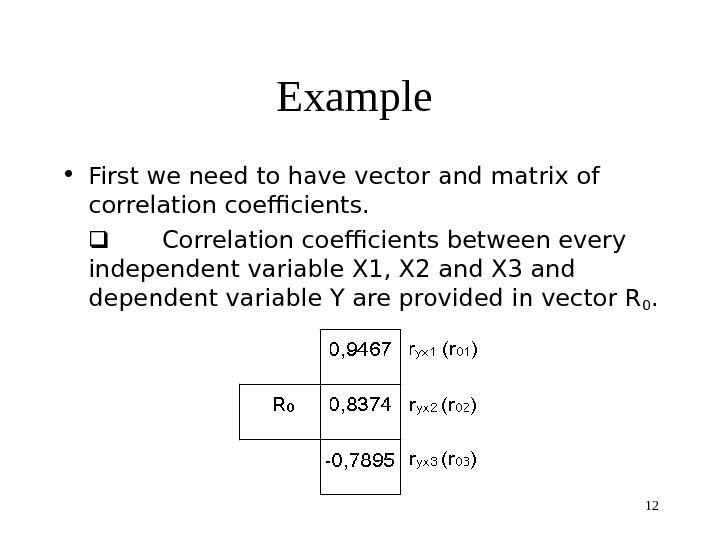12 Example • First we need to have vector and matrix of correlation coefficients. Correlation coefficients between every independent variable X 1, X 2 and X 3 and dependent variable Y are provided in vector R 0.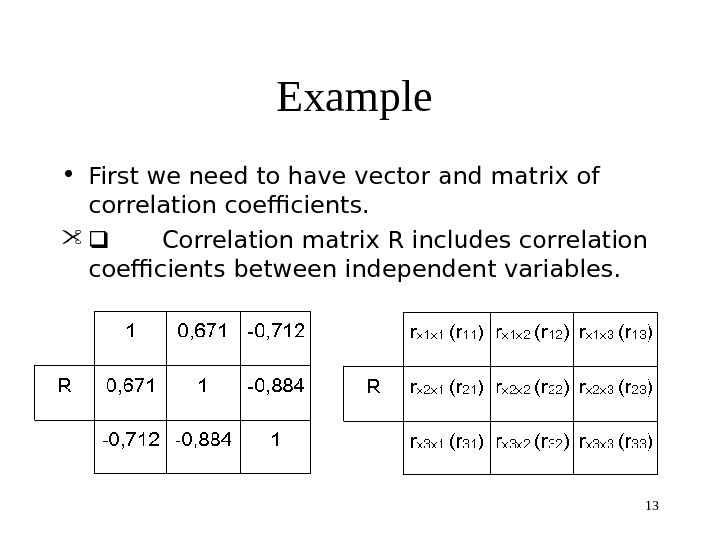13 Example • First we need to have vector and matrix of correlation coefficients. Correlation matrix R includes correlation coefficients between independent variables.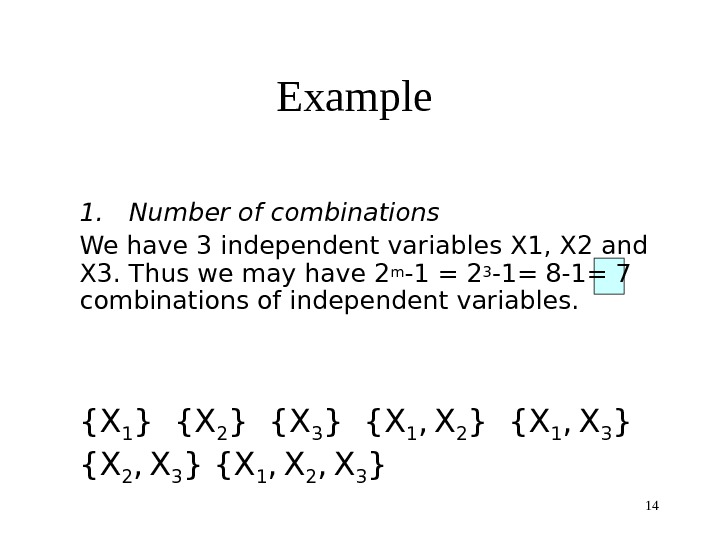14 Example 1. Number of combinations We have 3 independent variables X 1, X 2 and X 3. Thus we may have 2 m -1 = 2 3 -1= 8 -1= 7 combinations of independent variables. {X 1 } {X 2 } {X 3 } {X 1 , X 2 } {X 1 , X 3 } {X 2 , X 3 } {X 1 , X 2 , X 3 }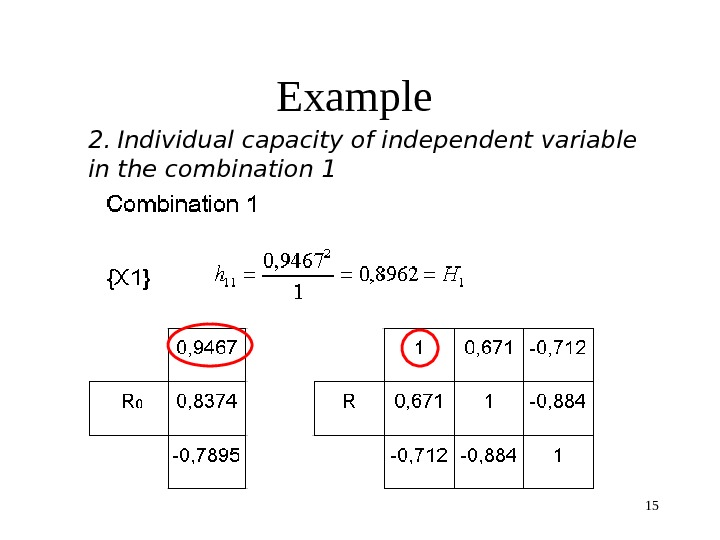15 Example 2. Individual capacity of independent variable in the combination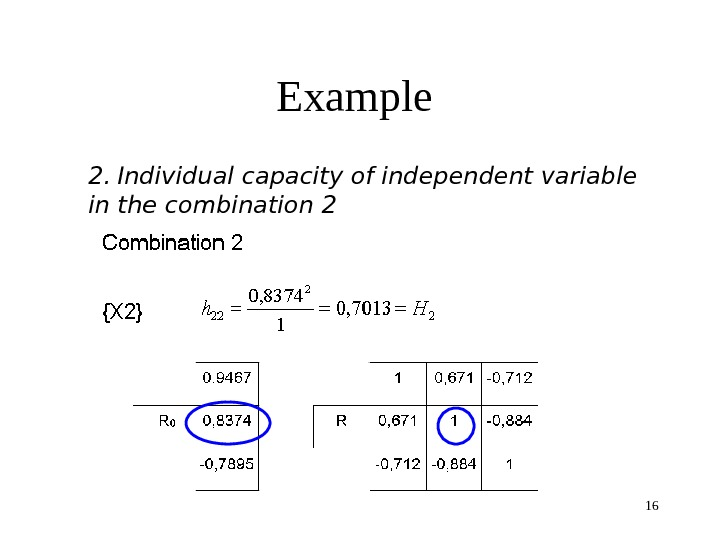16 Example 2. Individual capacity of independent variable in the combination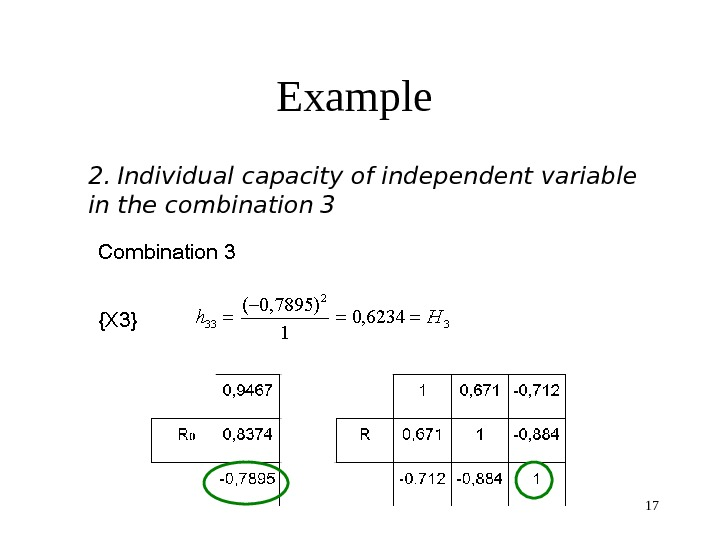17 Example 2. Individual capacity of independent variable in the combination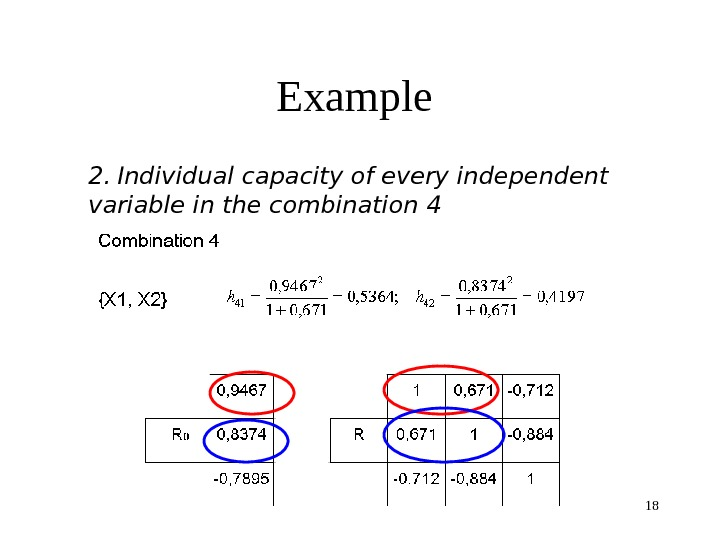18 Example 2. Individual capacity of every independent variable in the combination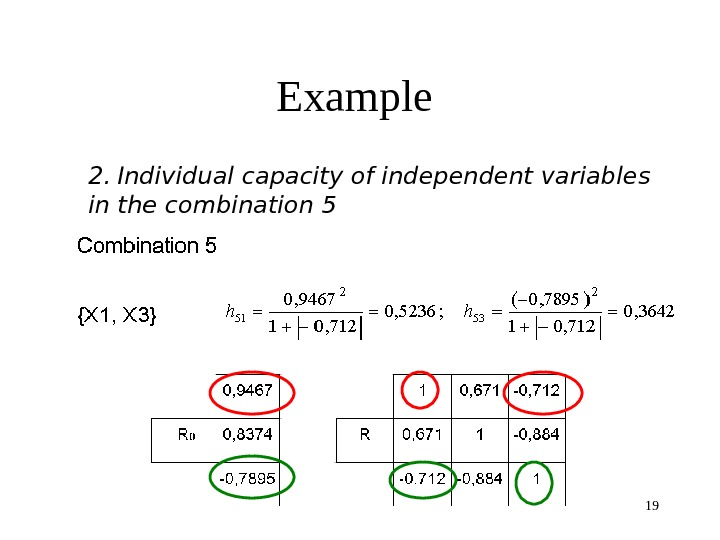19 Example 2. Individual capacity of independent variable s in the combination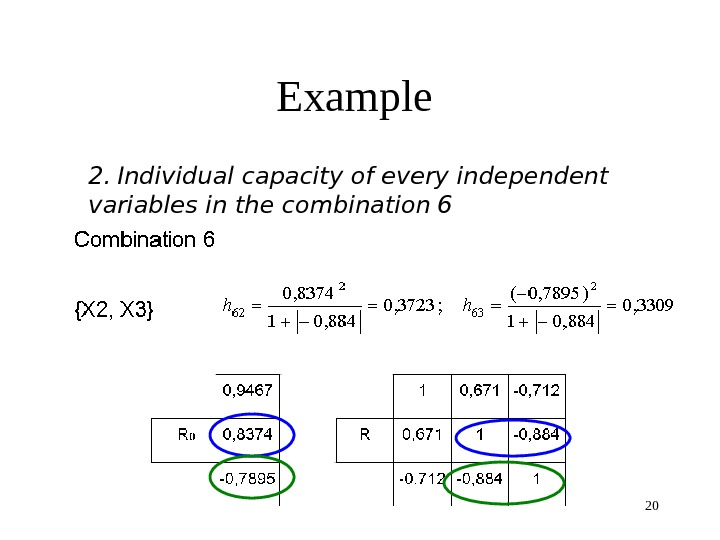20 Example 2. Individual capacity of every independent variable s in the combination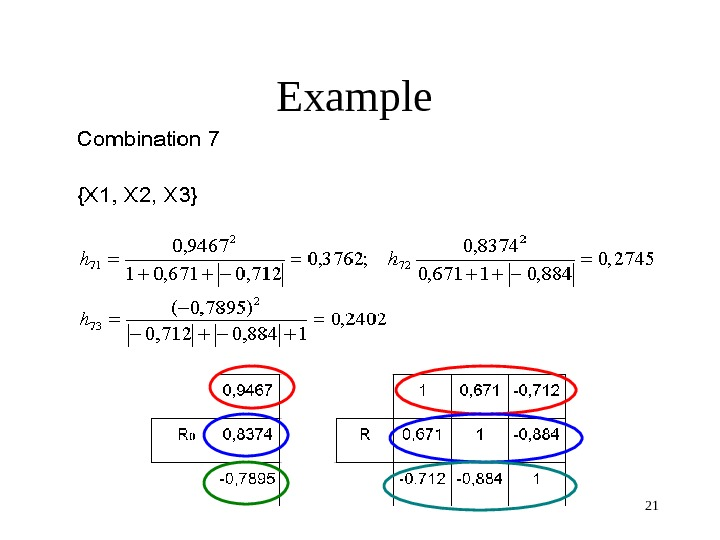21 Example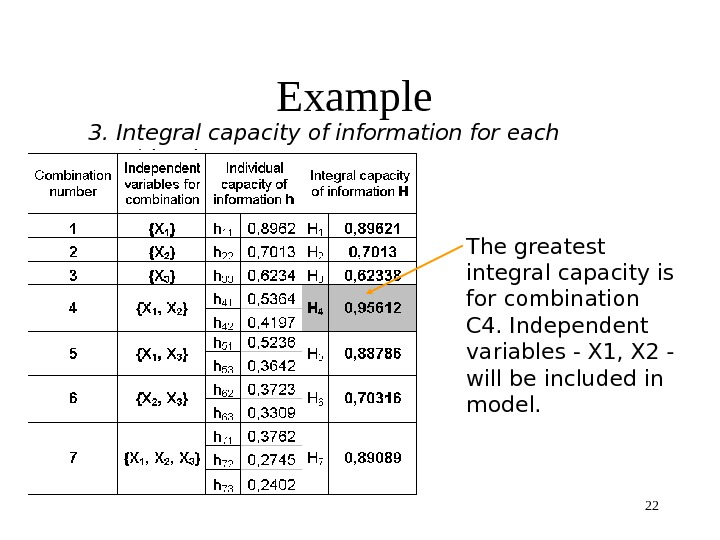22 Example 3. Integral capacity of information for each combination The greatest integral capacity is for combination C 4. Independent variables — X 1, X 2 — will be included in model.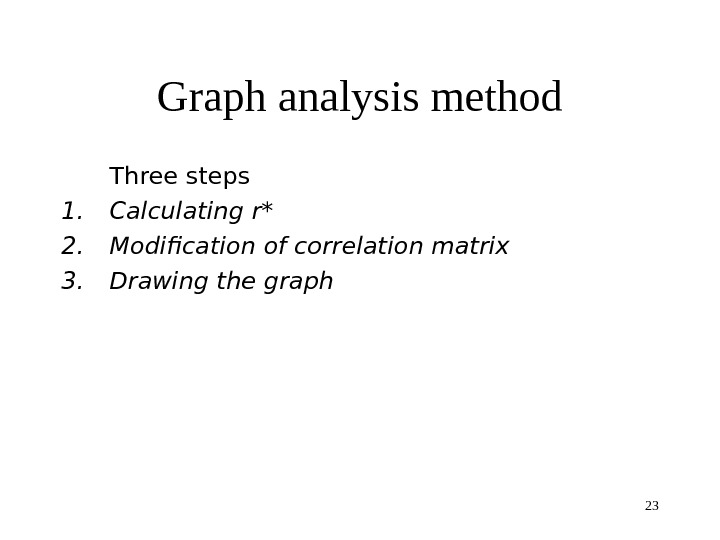23 Graph analysis method Three steps 1. Calculating r* 2. Modification of correlation matrix 3. Drawing the graph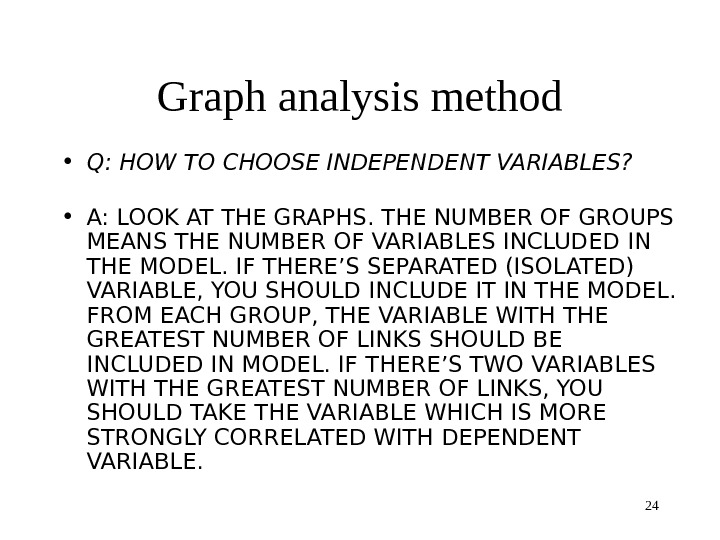24 Graph analysis method • Q: HOW TO CHOOSE INDEPENDENT VARIABLES? • A: LOOK AT THE GRAPHS. THE NUMBER OF GROUPS MEANS THE NUMBER OF VARIABLES INCLUDED IN THE MODEL. IF THERE’S SEPARATED (ISOLATED) VARIABLE, YOU SHOULD INCLUDE IT IN THE MODEL. FROM EACH GROUP, THE VARIABLE WITH THE GREATEST NUMBER OF LINKS SHOULD BE INCLUDED IN MODEL. IF THERE’S TWO VARIABLES WITH THE GREATEST NUMBER OF LINKS, YOU SHOULD TAKE THE VARIABLE WHICH IS MORE STRONGLY CORRELATED WITH DEPENDENT VARIABLE.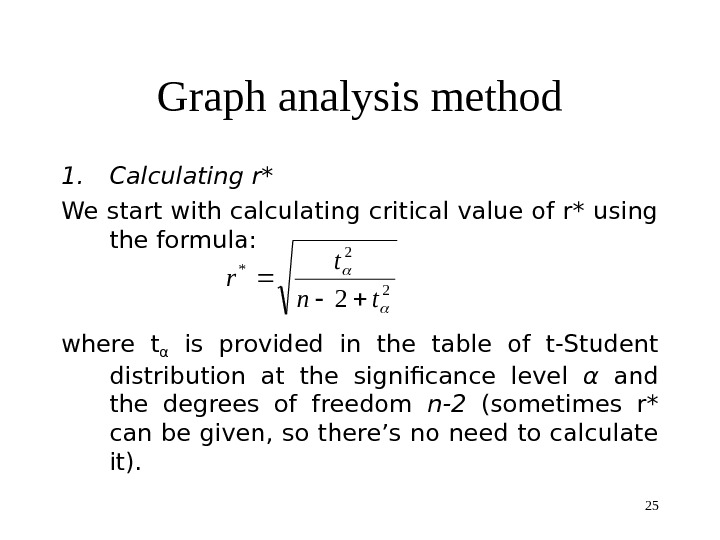25 Graph analysis method 1. Calculating r* We start with calculating critical value of r* using the formula: where tα is provided in the table of t-Student distribution at the significance level α and the degrees of freedom n-2 (sometimes r* can be given, so there’s no need to calculate it). 2 2 * 2 tn t r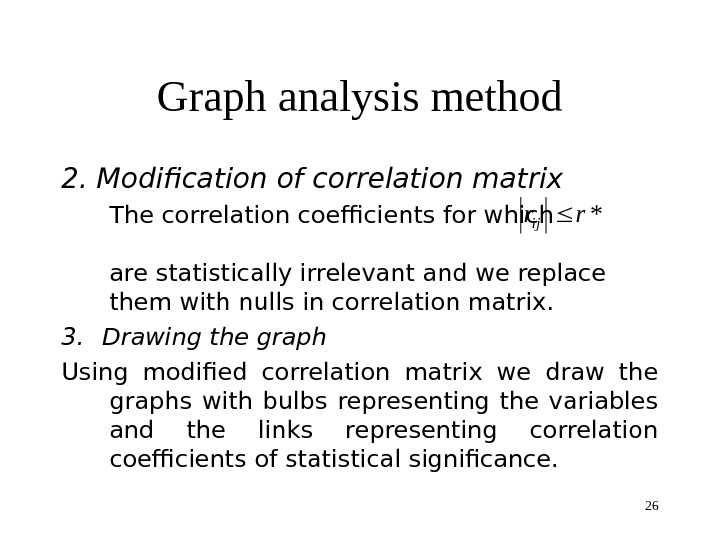26 Graph analysis method 2. Modification of correlation matrix The correlation coefficients for which are statistically irrelevant and we replace them with nulls in correlation matrix. 3. Drawing the graph Using modified correlation matrix we draw the graphs with bulbs representing the variables and the links representing correlation coefficients of statistical significance. *rrij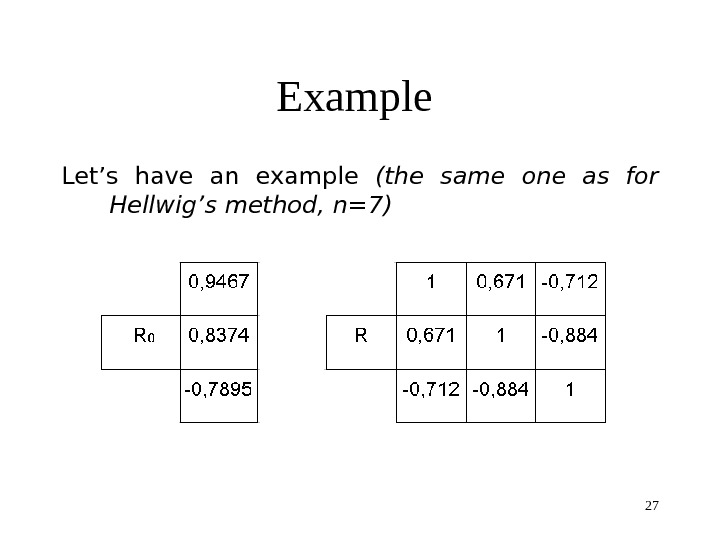27 Example Let’s have an example (the same one as for Hellwig’s method, n=7)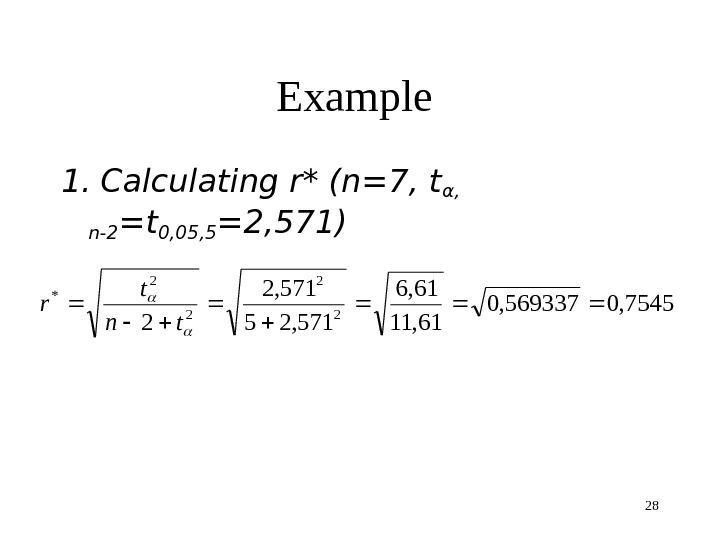28 Example 1. Calculating r* (n=7, tα, n-2 =t 0, 05, 5 =2, 571) 7545, 0569337, 0 61, 11 61, 6 571, 25 571, 2 2 2 * tn t r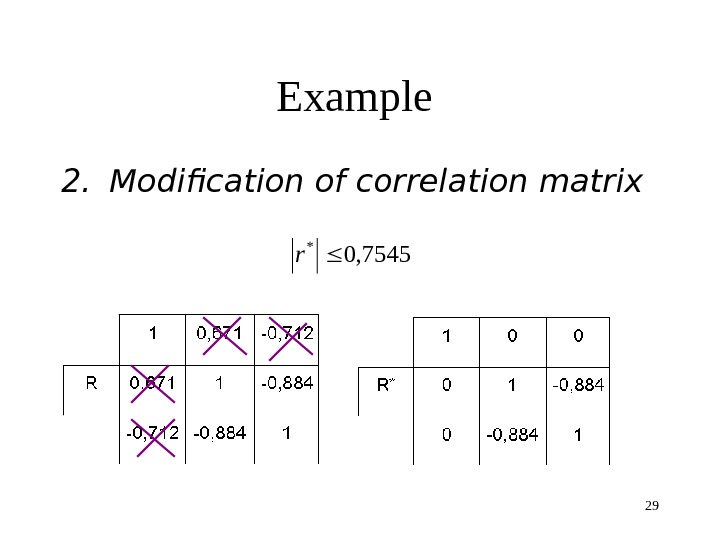29 Example 2. Modification of correlation matrix 7545, 0 * r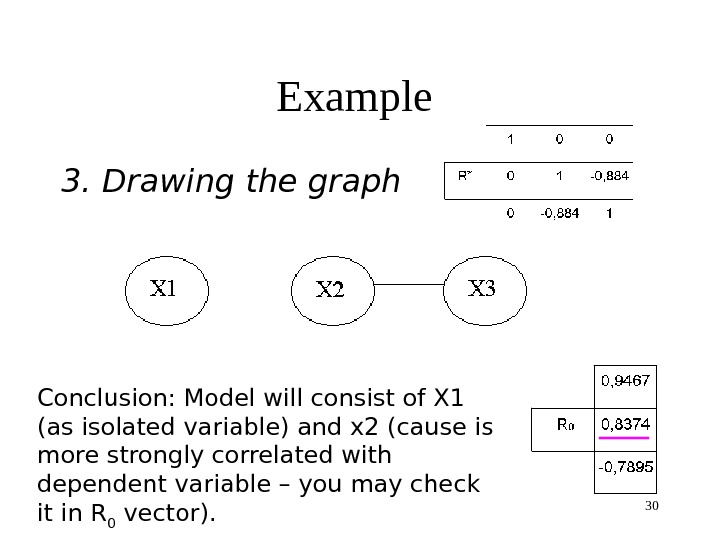30 Example 3. Drawing the graph Conclusion: Model will consist of X 1 (as isolated variable) and x 2 (cause is more strongly correlated with dependent variable – you may check it in R 0 vector).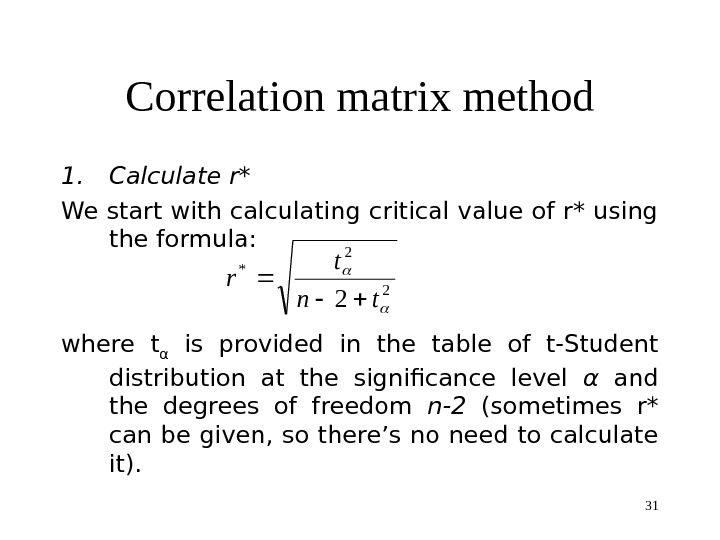31 Correlation matrix method 1. Calculate r* We start with calculating critical value of r* using the formula: where t α is provided in the table of t-Student distribution at the significance level α and the degrees of freedom n-2 (sometimes r* can be given, so there’s no need to calculate it). 2 2 * 2 tn t r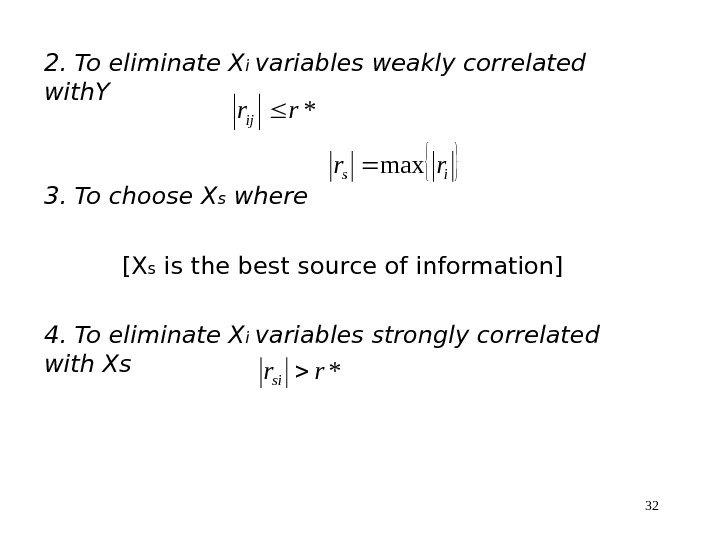322. To eliminate X i variables weakly correlated with. Y 3. To choose X s where [X s is the best source of information] 4. To eliminate X i variables strongly correlated with Xs*rrij isrrmax *rrsi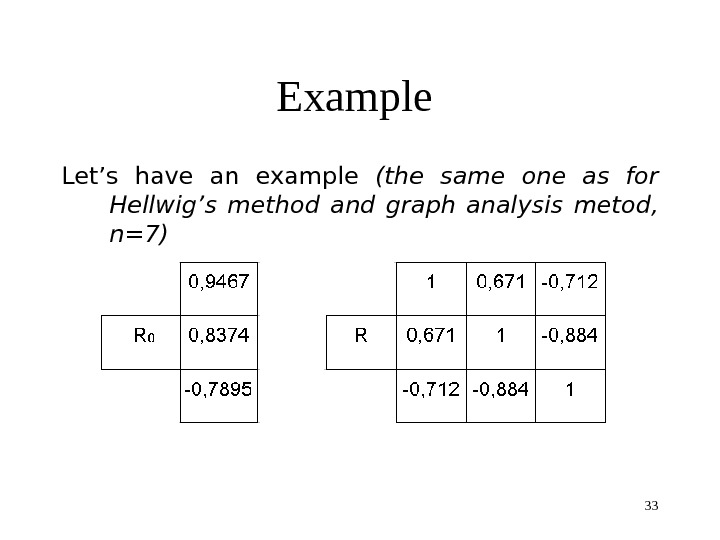33 Example Let’s have an example (the same one as for Hellwig’s method and graph analysis metod , n=7)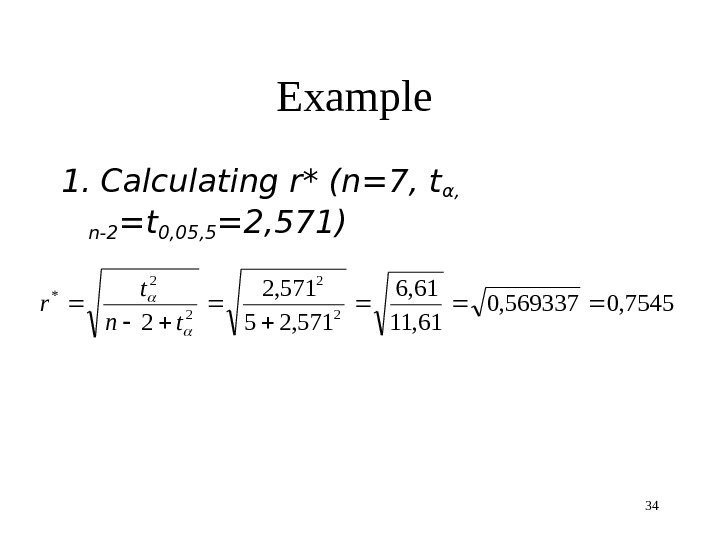34 Example 1. Calculating r* (n=7, tα, n-2 =t 0, 05, 5 =2, 571) 7545, 0569337, 0 61, 11 61, 6 571, 25 571, 2 2 2 * tn t r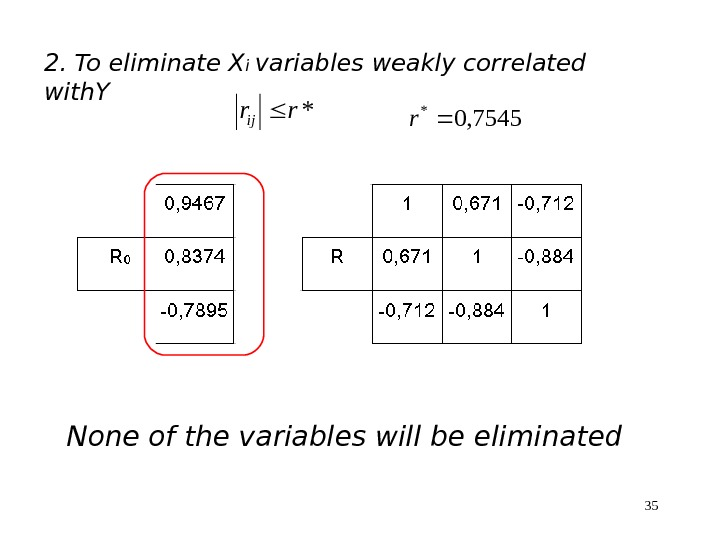352. To eliminate X i variables weakly correlated with. Y*rrij None of the variables will be eliminated 7545, 0 * r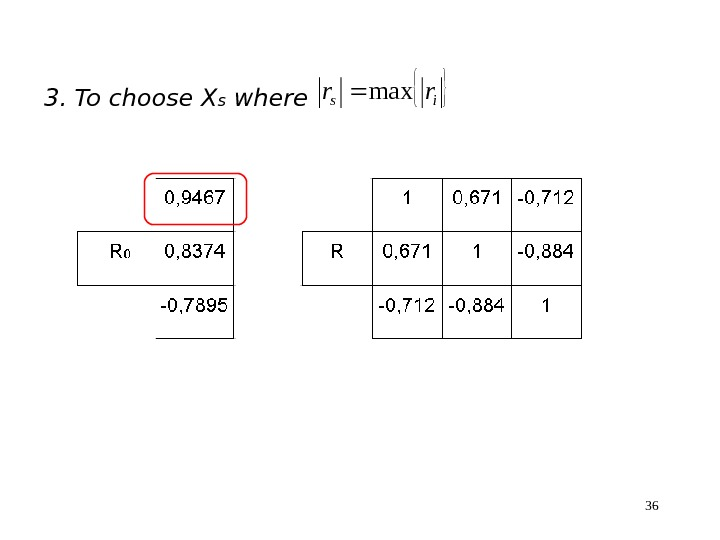363. To choose X s where isrrmax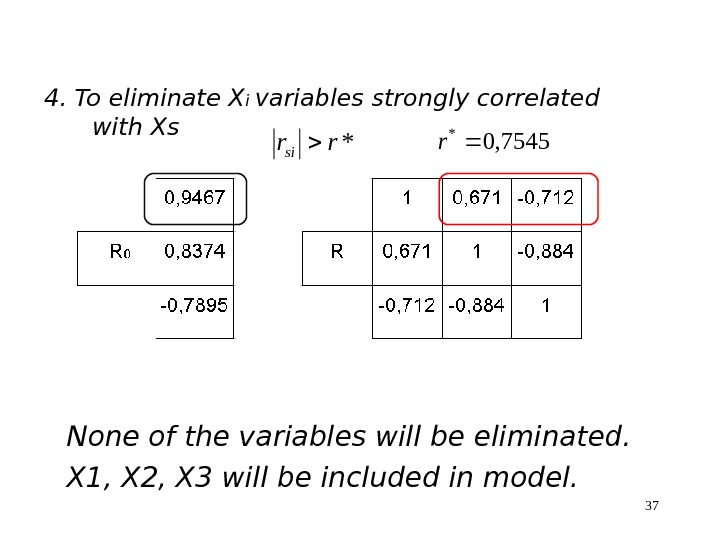374. To eliminate X i variables strongly correlated with Xs*rrsi 7545, 0 * r None of the variables will be eliminated. X 1, X 2, X 3 will be included in model.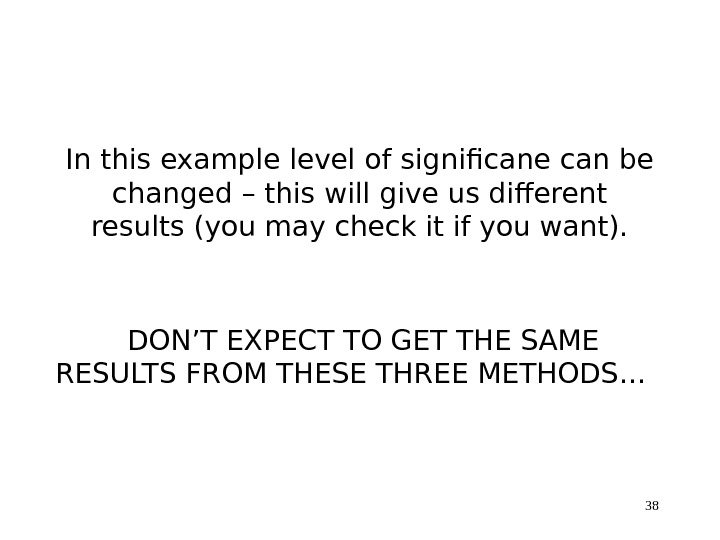38 In this example level of significane can be changed – this will give us different results (you may check it if you want). DON’T EXPECT TO GET THE SAME RESULTS FROM THESE THREE METHODS…

Зарегистрируйтесь, чтобы просмотреть полный документ!
РЕГИСТРАЦИЯ